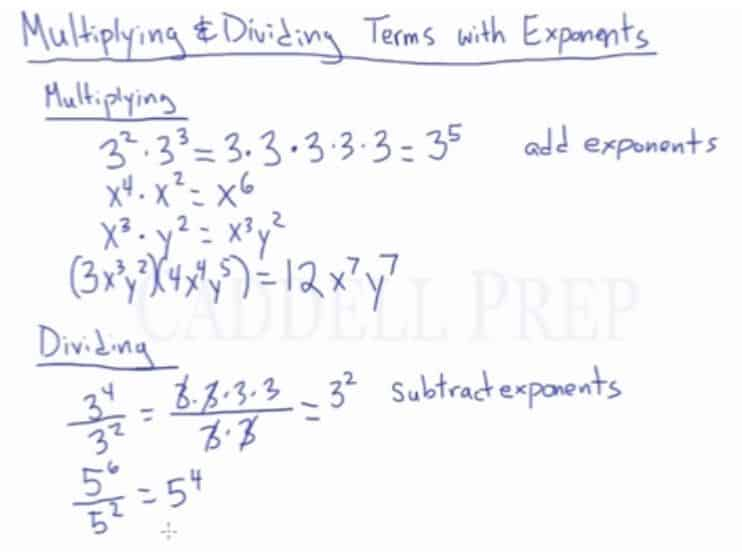In this video, we are going to look at how to multiply and divide terms with exponents. After you finish this lesson, view all of our Pre-Algebra lessons and practice problems. After you finish this lesson, view all of our Algebra 1 lessons and practice problems.

## Example of Multiplying Terms with Exponents

To solve the following:$3^2 \times 3^3$
We add the exponents when the bases are the same, to get$3^5$
To solve something more complex such as$(3x^3y^2)(4x^4y^5)$
we multiply the constants first, then we multiply the terms with the same base to get$12x^7y^7$
To solve the following:$3^4 \div 3^2$
we subtract the exponents when the bases are the same, to get$3^2$

Other examples:

Multiplying Terms that have Exponents and the Same Base$x^a \times x^b = x^{a+b}$$3^9 \times 3^4 = 3^{9+4} = 3^{13}$

Dividing Terms that have Exponents and the Same Base$x^a \div x^b = x^{a-b}$$3^9 \div 3^4 = 3^{9-4} = 3^{5}$

## Examples of Properties of Exponents

### Example 1$(9a^{3}b^{2}c{4})(3a^{4}b^{6}c^{3})$

First, we simply multiply the constants$9 \times 3 = 27$

Then, we multiply the terms with the same base$a^{3+4}=a^{7}$$b^{2+6}=b^{8}$$c^{4+3}=c^{7}$

Now, we have:$27a^{27}b^{8}c^{7}$

### Example 2$18x^{8} \div 6x^{2}$

First we have to divide the constants$\dfrac{18x^{8}}{ 6x^{2}}= \dfrac{ 6x^{8}}{ x^{2}}$

In dividing, we just have to subtract the exponents$6x^{8-2}$

Now, we have:$6x^{6}$

## Video-Lesson Transcript

In this video, we are going to look at how to multiply and divide terms with exponents.

Let’s go to Multiplication first.

We have$3^2 \times 3^3$

So we have$3^2 \times 3^3 = 3 \times 3 \times 3 \times 3 \times 3$$= 3^5$

Another example,$x^4 \times x^2$

So instead of writing it all out, we just have to add the exponents.

In Multiplying, we just have to add the exponents and will have$4 + 2 = 6$

The final answer is$x^6$

Next, we have$x^3 x y^2$.

They have different base so the final answer is just$x^3 y^2$

Let’s move on to a more complicated one.$\big(3 x^3 y^2\big) \big(4 x^4 y^5\big)$

You can multiply this simply by multiplying the two coefficients first$3 \times 4 = 12$

Then, the two with the similar base$x^{3 + 4} = x^7$

We will have$x^7$

Then$y^{2 + 5} = y^7$

And the answer is$y^7$

Our final answer is$12 x^7 y^7$

Now, let’s move on to Division.$\dfrac{3^4}{3^2}$

So$= \dfrac{3 \times 3 \times 3 \times 3}{3 \times 3}$

We’ll cancel out and will come up with$= 3^2$

So in Dividing, we just have to subtract the exponents.

Another example is$\dfrac{5^6}{5^2}$

It should be$5^{6 - 2} = 5^4$

And we’ll have$5^4$